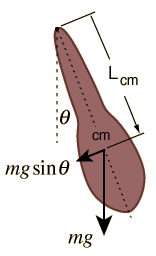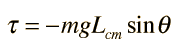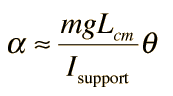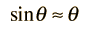# Physical PendulumHanging objects may be made to oscillate in a manner similar to a simple pendulum. The motion can be described by "Newton's 2nd law for rotation":where the torque isand the relevant moment of inertia is that about the point of suspension. The resulting equation of motion is:for small angles whereShow

This is identical in form to the equation for the simple pendulum and yields a period:Calculation Compare to simple pendulum

 Rod pendulum Circular geometries Combinations
Index

Periodic motion concepts

 HyperPhysics***** Mechanics R Nave
Go Back

# Physical Pendulum CalculationThe period is not dependent upon the mass, since in standard geometries the moment of inertia is proportional to the mass.

For small displacements, the period of the physical pendulum is given byFor Isupport = kg m2 m = kg g = m/s2 Lcm = cm the period is T = s
 Rod pendulum Circular geometries Combinations
Index

Periodic motion concepts

 HyperPhysics***** Mechanics R Nave
Go Back